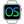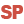ONLINE HELP WINDEV, WEBDEV AND WINDEV MOBILEVersion: 15161718192021222324252627
 Home |Sign in | EnglishHelpWLanguageWLanguage syntaxType of dataAdvanced typesRectanglePresentationExampleWLanguage properties that can be used with the Rectangle typeAssigning a Rectangle variableAssigning an array of RectanglesComparison and serializationNotesSee alsoAlias typeBufferCharacter stringsConverting C typesCurrenciesDateDateTimeDifferent types of dataDurationJSONNumericOperations available for the dates, times and durations, ...RealsRectangleThe different types of integersTimeUUIDVariantYAML
• WLanguage properties that can be used with the Rectangle type
• Assigning a Rectangle variable
• Assigning an array of Rectangles
• Comparison and serialization
• Notes
WINDEVWEBDEVWINDEV MobileOthersRectangle (Type of variable)
In french: Rectangle
A Rectangle variable is a structured type that is used to represent a rectangle (X, Y, Width, Height) in 2 dimensions.Versions 24 and laterThis type of variable is now available in Android Widget mode.This type of variable is now available for Android applications.This type of variable is now available for Java applications.New in version 24This type of variable is now available in Android Widget mode.This type of variable is now available for Android applications.This type of variable is now available for Java applications.This type of variable is now available in Android Widget mode.This type of variable is now available for Android applications.This type of variable is now available for Java applications.
Example
MyRectangle is Rectangle
MyRectangle.X = 280
MyRectangle.Y = 54
MyRectangle.Width = 50
MyRectangle.Height = 150
Syntax

Declaring and initializing a rectangle

<Rectangle Name> is Rectangle
<Rectangle Name>:
Name of the Rectangle variable to declare.
Remarks

WLanguage properties that can be used with the Rectangle type

The initial value of a rectangle is set to (0,0,0,0).
The following properties can be used to handle a Rectangle variable:
Property name Effect Height Height of the rectangle. This coordinate corresponds to a signed 4-byte integer. Width Width of the rectangle. This coordinate corresponds to a signed 4-byte integer. X X-coordinate of the top left point. This coordinate corresponds to a signed 4-byte integer. Y Y-coordinate of the top left point. This coordinate corresponds to a signed 4-byte integer.

Assigning a Rectangle variable

A Rectangle variable can be assigned with:
• another Rectangle variable.
For example:
MyRectangle is Rectangle
MyRectangle.X = 280
MyRectangle.Y = 54
MyRectangle.Width = 50
MyRectangle.Height = 150
MyRectangle1 is Rectangle = MyRectangle
• an array of four values that can be converted into integer.
For example:
MyRectangle is Rectangle
MyRectangle = [280, 54, 50, 150]
• two Point variables (corresponding respectively to the upper-left corner and to the lower-right corner of the rectangle).
For example:
MyRectangle is Rectangle
MyRectangle = [[280, 54], [330, 204]]

Assigning an array of Rectangles

An array of rectangles can be assigned with:
• an array of values containing rectangles.
For example:
ArrayOfRectangles is array of Rectangles
ArrayOfRectangles = [MyRectangle1, MyRectangle2]
• an array of four values that can be converted into integer.
For example:
ArrayOfRectangles is array of Rectangles
ArrayOfRectangles = [[1,2,3,4], [5,6,7,8]

Comparison and serialization

• Comparison: The comparison operators = and <> are available.
• Serialization: The binary, XML and JSON serializations are available.
Caution: During an XML serialization, the xmlAttribute constant cannot be used on a Rectangle class member or on a Rectangle structure member.

Notes

• A Rectangle variable can be stored in a variant.
• A Rectangle variable is never null:
• The "RectangleVariable = NULL" condition always returns False.
• The "RectangleVariable <> NULL" condition always returns True.
• You cannot reference a Rectangle variable.
The "RectangleVariable2 <- RectangleVariable1" syntax triggers an error.
• You cannot force the copy of a Rectangle variable.
The "RectangleVariable2 <= RectangleVariable1" syntax triggers an error.
• You have the ability to add a Point variable and a Rectangle variable. This operation is used to perform a relocation of the rectangle.
• You have the ability to subtract a Point variable to a Rectangle variable. This operation is used to perform a relocation of the rectangle.
• The Rectangle variables can be passed to the system APIs.
Minimum version required
• Version 21